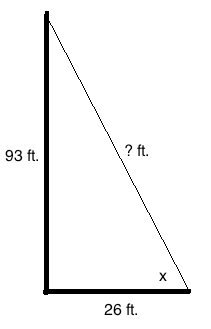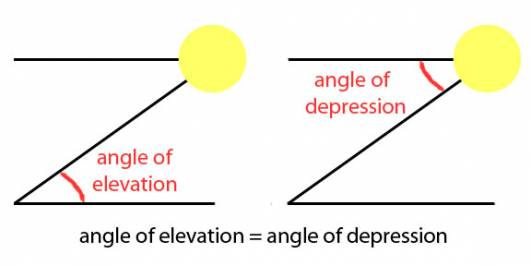# A tree which is 93 feet tall casts a shadow of 26 feet. Find the angle of elevation of the sun?

## 1 Answers

David Shabazi answered

Based on the information given, just draw it all out. Then for information you don't know, leave them blank. Or put a variable next to them so they resemble some numeric value.And to recap, here is the difference between an angle of elevation and depression:Ignore the part that says "angle of elevation = angle of depression".

So we're trying to find the angle of elevation, or x, in this case. Well, do you recall that using tangent to find degrees is opposite over adjacent?

Soh Cah Toa = Sine: Opposite/hypotenuse, Cosine: Adjacent/hypotenuse, Tangent: Opposite/adjacent.

On the opposite side of x, we see the line that represents 93 feet. And adjacent to x, we see a line that resembles 26 feet. So if tangent = opposite/adjacent, that must mean:

tan x = 93/26

Simplify 93/26, which will give roughly 3.58.

tan x = 3.58

Take the tangent inverse of both sides (which is tan^-1 (x)). This will cancel out the tangent on the left side of the function, giving you x.

x = tan^-1 (3.58)

From them on, you have to use a calculator to determine what tan^-1 (3.58) is. Once you get the exact answer, that is your angle of elevation.

2 People thanked the writer.
View all 4 Comments
John McCann commented
I have been waiting for you to answer this two days now David!

Did you think an old man like me wanted to get off his duff and take down a trig book?
David Shabazi commented
Sorry, I was busy the past couple of days and couldn't find time to answer math questions as frequently as I used to. But I'll try to answer more frequently from now on.
John McCann commented
Good!

The older I get the lazier I get.

My are is biology and little of that here for sure.

## Answer Question